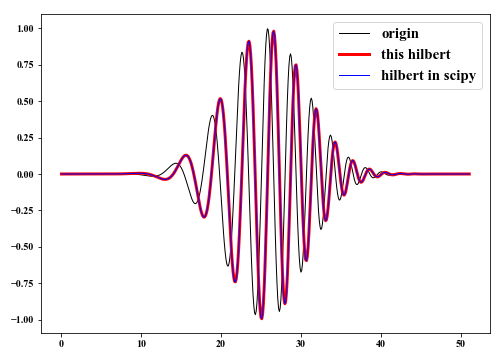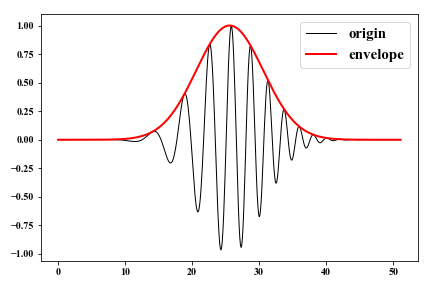Firstly, compute the FFT of real signal $x(n)$ $$X(k) = \mathscr{F}[x(n)]=\sum_{n=0}^{N-1}x(n)e^{-\frac{i2\pi kn}{N}} \tag{1}.$$

Let the FFT of $h(n)$ be $H(k)$ to yield
$$H(k)= \begin{cases} &X(k), &\text{ for } k=0 \\ &2X(k), &\text{ for } k=1, 2, \cdots, \frac{N}{2}-1 \\ &0, &\text{ for } k=\frac{N}{2}, \frac{N}{2}+1,\cdots, N-1 \end{cases} \tag{2}.$$

The hilbert transform $h(n)$ of the real signal $x(n)$ is given by
$$h(n) = \mathscr{I} \{ \mathscr{F}^{-1}[H(k)] \} \tag{3}.$$ $h(n)$ and $x(n)$ are the imaginary and real parts of signals, respectively. Here, we implement the $Hilbert \ transform$ using Python and compare it with that computed with $hiltert$ method in $scipy.signal$.

  1 2 3 4 5 6 7 8 9 10 11 12 13 14 15 16 17 18 19 20 21 22 23  import numpy as np import matplotlib.pyplot as plt from scipy.signal import hilbert n = 512 dt = 0.1 t = np.arange(n) * dt x = np.sin(t**2/25) * np.exp(-(t-t[n//2])**2/50) fx = np.fft.fft(x) fh = fx.copy() fh[1: n//2] = 2 * fx[1: n//2] fh[n//2:] = 0 h1 = np.fft.ifft(fh).imag h2 = hilbert(x).imag plt.figure(figsize=(10, 6)) plt.plot(t, x, 'k', lw=1, label='origin') plt.plot(t, h1, 'r', lw=3, label='this hilbert') plt.plot(t, h2, 'b', lw=1, label='hilbert in scipy') plt.legend(fontsize=15) plt.tight_layout() plt.show()The envelope of a signal can be calculated using $Hilbert \ transform$ with
$$E(n) =\sqrt{x^2(n)+h^2(n)} \tag{4}.$$

 1 2 3 4 5 6 7  e = (x**2+h1**2) ** 0.5 plt.figure(figsize=(8, 5)) plt.plot(t, x, 'k', lw=1, label='origin') plt.plot(t, e, 'r', lw=2, label='envelope') plt.legend(fontsize=15) plt.tight_layout() plt.show()